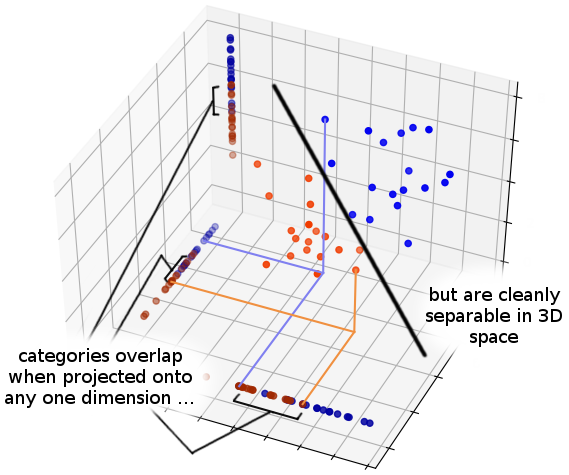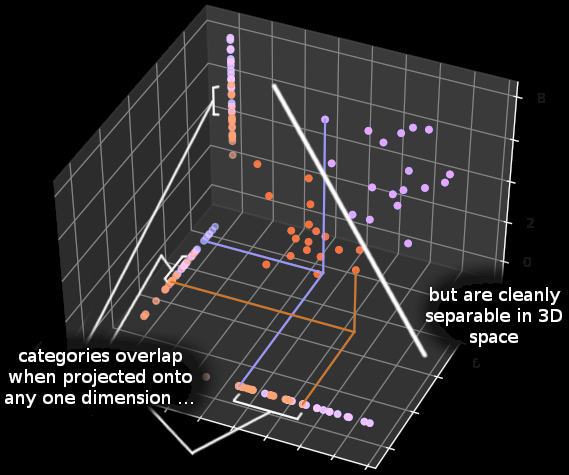# The Univariate Fallacy

(A standalone math post that I want to be able to link back to later/​elsewhere)

There’s this statistical phenomenon where it’s possible for two multivariate distributions to overlap along any one variable, but be cleanly separable when you look at the entire configuration space at once. This is perhaps easiest to see with an illustrative diagram—The denial of this possibility (in arguments of the form, “the distributions overlap along this variable, therefore you can’t say that they’re different”) is sometimes called the “univariate fallacy.” (Eliezer Yudkowsky proposes “covariance denial fallacy” or “cluster erasure fallacy” as potential alternative names.)

Let’s make this more concrete by making up an example with actual numbers instead of just a pretty diagram. Imagine we have some datapoints that live in the forty-dimensional space {1, 2, 3, 4}⁴⁰ that are sampled from one of two probability distibutions, which we’ll call and .

For simplicity, let’s suppose that the individual variables x₁, x₂, … x₄₀—the coördinates of a point in our forty-dimensional space—are statistically independent and identically distributed. For every individual , the marginal distribution of is—

And for

If you look at any one -coördinate for a point, you can’t be confident which distribution the point was sampled from. For example, seeing that x₁ takes the value 2 gives you a 74 (= 1.75) likelihood ratio in favor of that the point having been sampled from rather than , which is log₂(7/​4) ≈ 0.807 bits of evidence.

That’s … not a whole lot of evidence. If you guessed that the datapoint came from based on that much evidence, you’d be wrong about 4 times out of 10. (Given equal (1:1) prior odds, an odds ratio of 7:4 amounts to a probability of (7/​4)/​(1 + 74) ≈ 0.636.)

And yet if we look at many variables, we can achieve supreme, godlike confidence about which distribution a point was sampled from. Proving this is left as an exercise to the particularly intrepid reader, but a concrete demonstration is probably simpler and should be pretty convincing! Let’s write some Python code to sample a point ∈ {1, 2, 3, 4}⁴⁰ from

import random

def a():
return random.sample(
*4 +  # 1/4
*7 +  # 7/16
*4 +  # 1/4
,     # 1/16
1
)

x = [a() for _ in range(40)]
print(x)


Go ahead and run the code yourself. (With an online REPL if you don’t have Python installed locally.) You’ll probably get a value of x that “looks something like”

[2, 1, 2, 2, 1, 1, 2, 2, 1, 2, 1, 4, 4, 2, 2, 3, 3, 1, 2, 2, 2, 4, 2, 2, 1, 2, 1, 4, 3, 3, 2, 1, 1, 3, 3, 2, 2, 3, 3, 4]


If someone off the street just handed you this without telling you whether she got it from or , how would you compute the probability that it came from ?

Well, because the coördinates/​variables are statistically independent, you can just tally up (multiply) the individual likelihood ratios from each variable. That’s only a little bit more code—

import logging

logging.basicConfig(level=logging.INFO)

def odds_to_probability(o):
return o/(1+o)

def tally_likelihoods(x, p_a, p_b):
total_odds = 1
for i, x_i in enumerate(x, start=1):
lr = p_a[x_i-1]/p_b[x_i-1]  # (-1s because of zero-based array indexing)
logging.info(“x_%s = %s, likelihood ratio is %s”, i, x_i, lr)
total_odds *= lr

print(
odds_to_probability(
tally_likelihoods(
x,
[1/​4, 7/​16, 1/​4, 1/​16],
[1/​16, 1/​4, 7/​16, 1/​4]
)
)
)


If you run that code, you’ll probably see “something like” this—

INFO:root:x_1 = 2, likelihood ratio is 1.75
INFO:root:x_2 = 1, likelihood ratio is 4.0
INFO:root:x_3 = 2, likelihood ratio is 1.75
INFO:root:x_4 = 2, likelihood ratio is 1.75
INFO:root:x_5 = 1, likelihood ratio is 4.0
[blah blah, redacting some lines to save vertical space in the blog post, blah blah]
INFO:root:x_37 = 2, likelihood ratio is 1.75
INFO:root:x_38 = 3, likelihood ratio is 0.5714285714285714
INFO:root:x_39 = 3, likelihood ratio is 0.5714285714285714
INFO:root:x_40 = 4, likelihood ratio is 0.25
0.9999936561215961


Our computed probability that came from has several nines in it. Wow! That’s pretty confident!